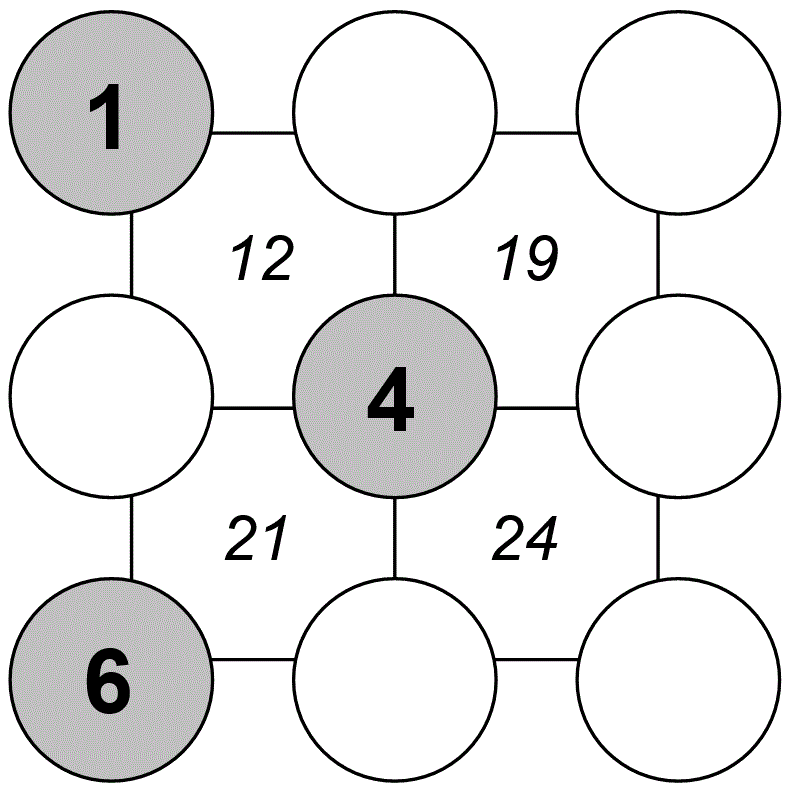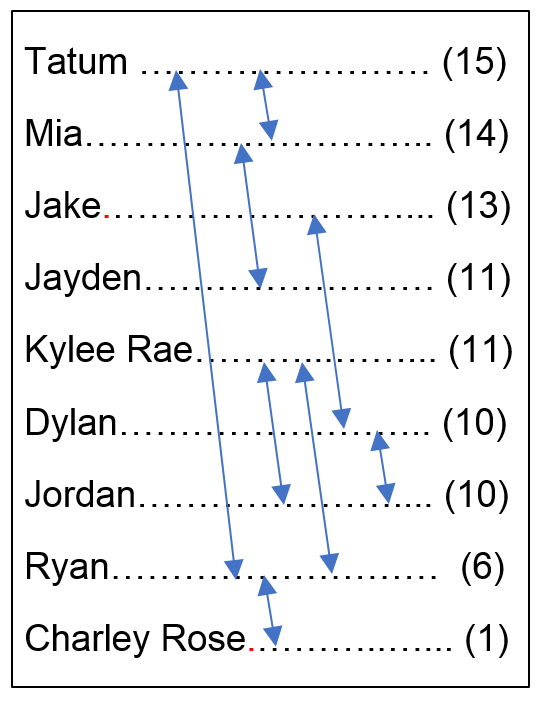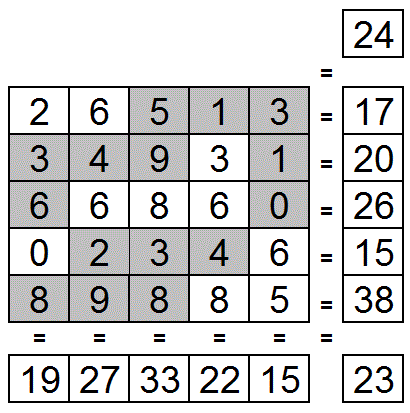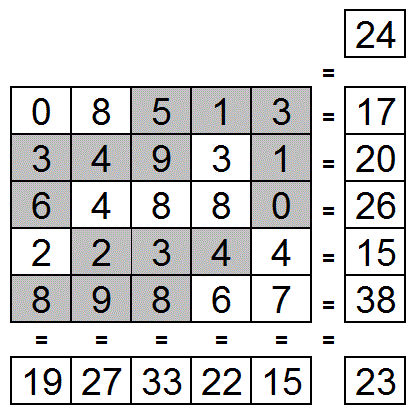Select Page

Each month, a new set of puzzles will be posted.  Come back next month for the solutions and a new set of puzzles, or subscribe to have them sent directly to you.

## MIND-Xpander maths puzzles

1. How many hours will a car traveling at 45 mph take to catch up with another car travelling at 30 mph if the slower car starts one hour before the faster car?
2. A student needs at least 95% average to receive a grade A. On the first 3 tests, the student averaged 92%. What must the student average on the last 2 tests to receive a grade of A?
3. Using all the digits from 1 to 9, can you form three, three-digit numbers. so that the third number is three times greater than the first and the second number equals the third minus the first? (Example: 219, 438, 657 and there are several additional solutions)

## SQUARE-Sums puzzles

The four numbers outside the nine circles represent the sum of the numbers in the four surrounding circles. The numbers in the circles can only be 1 to 9 and each number can be used only once. Three numbers have been provided to get you started. Find the remaining six numbers.## Feedback

There are more than one way of doing these puzzles and may well be more than one answer.  Please let me and others know what alternatives you find by commenting below.  We also welcome general comments on the subject and any feedback you'd like to give.

If you have a question that needs a response from me or you would like to contact me privately, please use the contact form.

## Get more puzzles!

If you've enjoyed doing the puzzles, consider ordering the books;

• Book One - 150+ of the best puzzles
• Book Two - 200+ with new originals and more of your favourites

Both in a handy pocket sized format.   Click here for full details.

## IND-Xpander Logic Problem

Can you determine their ages? – Tatum is nine years older than Ryan and Ryan is four years older that Charley Rose. Mia is three years older than Jayden and one year younger than Tatum. Jordan is one year younger than Kylee Rae and Kylee Rae is five years older than Ryan. Dylan is three years younger than Jake and the same age as Jordan. The sums of Kylee Rae’s and Jordan’s ages is 21.

Solution:

Given: Sum of Kylee’s (K) & Jordan’s (J) ages = 21.  Therefore, K + J = 21, or  K + (K-1) = 2K – 1 = 21, or 2K = 22 & K = 11.  So, Kylee Rae’s age is 11 and Jordan’s age is 10.  The other ages are then determined as shown in the diagram.## STAR-Sums Puzzles

The number within the five triangles of the star is equal to the sum of the three numbers in its surrounding circles. The number in the centre of the star is equal to the sum of the five numbers in its surrounding circles. The numbers in the circles can only be 0 to 9 and each number can be used only once. Three numbers have been provided to get you started. Find the remaining 7 numbers.## SQUARE-UP Puzzles

The missing numbers are between 0 & 9. The numbers in each row add up to the totals on the right, and the columns add up to the totals along the bottom. The sums of the diagonals are also given. Find the missing numbers? (There may be more than one solution.)